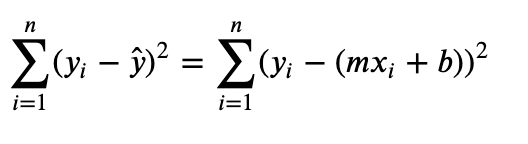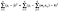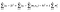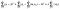# L1 vs L2 Regularization in Regression

Avoiding Overfitting in Regression ModelsPhoto by Michael Cox on Unsplash

In this post, I’m going to cover another very common technical interview question regarding regression that I, myself, could always brush up on:

Describing L1 vs L2 regularization methods in regression modeling.

When working with complex data, we tend to create complex models. And too complex is not always great. Overly complex models are what we call “overfit”, where they perform very well on training data, yet fall short in performance on unseen testing data. This also means high variance and low bias, which I delve into further in another post.

One way to adjust for overfitting in our loss function, and that is by penalization. By penalizing or “regularizing” large coefficients in our loss function, we make some (or all) of the coefficients smaller in an effort to desensitize the model to noise in our data.

The two popular forms of regularization are L1, AKA Lasso regression, and L2, AKA Ridge regression. With linear regression, we’ve seen how ordinary least squares (OLS) works in fitting to data: we square the residuals, the differences between actual values vs. predicted values, to get our Mean Squared Error (MSE). The smallest squared error, or least squares, is the best fit for the model.

Let’s take a look at the cost function for simple linear regression:The sum of the difference between our actual values, 𝑦, and predicted values, 𝑦̂, squared.

For multiple linear regression, the cost function would look something like this, where 𝑘 is the number of predictors or variables.The sum of the differences between our actual values, 𝑦, and predicted values, 𝑦̂, for each predictor, squared.

In multiple linear regression, as the number of predictors, 𝑘, increases, the model complexity increases. Increasing the number of predictors also increases the chance of multicollinearity occurring. Multicollinearity is when there is a strong correlation among the independent variables. To alleviate this, we add some form of penalty to this cost function. This will reduce the model complexity, help prevent from overfitting, possibly eliminate variables, and even reduce multicollinearity in the data.

## L2 — Ridge Regression

L2, or Ridge Regression, adds a 𝜆 penalty term to the square of the magnitude of the coefficients, 𝑚. This 𝜆 term is a hyperparameter, meaning it’s value is defined by you. You can see it at the end of the cost function here.L2 regularization penalty: 𝜆 x the sum of 𝑚(the magnitude of coefficients)-squared

With the 𝜆 penalty added, the 𝑚 coefficients are constrained and large coefficients penalize the cost function.

## L1 — Lasso Regression

L1, or Lasso Regression, is nearly the same thing except for one important detail- the magnitude of coefficients is not squared, it is just the absolute value.L1 regularization penalty: 𝜆 x the sum of m(the magnitude of coefficients)

With 𝑚 in absolute value at the end of the cost function here, some of the coefficients could be set exactly to zero, while others are just decreased towards zero. As some coefficients become zero, the effect of Lasso Regression is especially useful because it is estimating the cost and selecting the coefficients at the same time.

It is important to know that before you conduct either type of regularization, you should standardize your data to the same scale, otherwise the penalty will unfairly treat some coefficients.

I’m a recent Data Science graduate with a B.S. in Environmental Science. Currently seeking job opportunities. Constantly learning!

## More from Orin Conn

I’m a recent Data Science graduate with a B.S. in Environmental Science. Currently seeking job opportunities. Constantly learning!